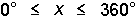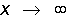﻿ Symbols

# Symbols

Top  Previous  Next
 Mathematicians use a large number of specialised symbols and FX Equation attempts to make accessing these symbols as easy as possible.  Any symbol that is not on the keyboard is entered using one of two methods.   Either A key is designated to be automatically replaced.  The two symbols that use this approach are °        uses the ` key (usually next to the 1 on the keyboard) ´        uses the * key.  The * symbol is often used to represent multiplication on computers.   or   A code is used and this code is automatically replaced with the appropriate symbol.  The code chosen has been chosen to be easily remembered and is usually a logical combination of characters.  For example   ≤                is built from < and = so the code is <= ≈                is built from two wavy lines so the code is ~ ~   Some codes are built from the word describing the symbol.   ∞        (infinity) the code is inf   Code                                          Symbol         < =                                                ≤ > =                                                ≥ < >  or  = /                                        ≠ = ~  or  ~=                                        ≅ ~ ~                                                ≈ - =                                                ≡ + -                                                ± - +                                                m *                                                      *, × or · / (followed by a space)                                ÷ `  or  o                                        � - >                                                → = >                                                ⇒ < = =                                        ⇐ dp                                                ·                 inf                                                ∞ tri                                                Δ ang                                                ∠ perp                                                ⊥ \$and                                                ∩ \$or                                                ∪ element                                        ∈ notelement                                        ∉ subset                                        ⊂ notsubset                                        ⊄ propersubset                                ⊆ es                                                ∅ prop                                                ∝ pd                                                ∂ tf                                                ∴ te                                                ∃ fa                                                ∀ nabla                                        ∇ au                                                Å CC                                                Complex Number Set Symbol ZZ                                                Integer Number Set Symbol QQ                                                Rational Number Set Symbol RR                                                Real Number Set Symbol NN                                                Natural Number Set Symbol _and                                                ∧, & or • _or                                                ∨ _not                                                ~ or ¬ _iff                                                ↔ or ≡ _imp                                                → or ⊃ _nor                                                ∇ _qed                                                ∴ Note: Some symbols have two codes.is entered as 0` <= x <= 360`is entered as x -> inf